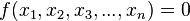Diophantine equation facts for kids

Kids Encyclopedia Facts

A Diophantine equation is an equation that only takes integer coefficients, and that can be written as$f(x_1, x_2, x_3, . . ., x_n) = 0$, where f is a polynomial. Diophantine analysis is a branch of mathematical analysis, concerned with such equations. Typical questions include:

1. Are there any solutions?
2. Are there any solutions beyond some that are easily found by inspection?
3. Are there finitely or infinitely many solutions?
4. Can all solutions be found in theory?
5. Can one in practice compute a full list of solutions?

Very often such problems were unresolved for centuries. With time, mathematicians came to understand their depth (in some cases), rather than treat them as puzzles. The equation is named after Diophantus of Alexandria, a mathematician, who described them first. The restriction on integer coefficients makes sense, when one is concerned about finding Divisors, or in the case of modular arithmetic. In everyday life, many equations solve problems where only whole numbers make sense: A product is composed of many parts, but only whole pieces can be produced.

Some diophantine equations are very famous. These include the Pythagorean triple, Fermat's Last Theorem and Pell's equation. Hilbert's tenth problem was to find an algorithm to decide, whether a given Diophantine equation has an integer solution.Diophantine equation Facts for Kids. Kiddle Encyclopedia.